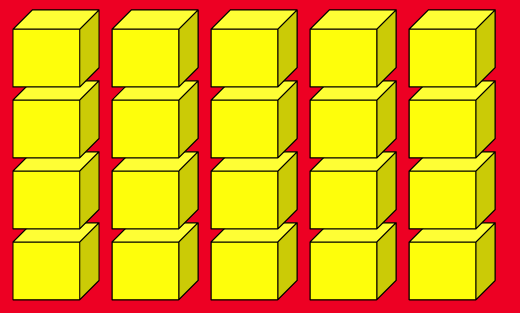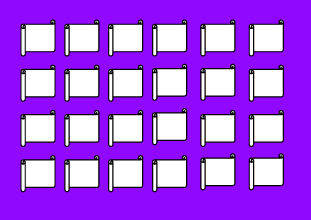## Wednesday, 3 September 2014

### The Rescue

The rescue from Team 3 PES on Vimeo.

we are lerning to create movies on the ipad.

## Monday, 1 September 2014

### my math

Write the multiplication equations for the following arrays:
1.5 x 4 = 20

2.2 x 3 = 21 3 x 7 =
3.4x6= 24

Write the multiplication equations for the following arrays:
1.5 x 4 = 20

2.2 x 3 = 21 3 x 7 =
3.4x6= 24

Write the multiplication equations for the following arrays:
1.5 x 4 = 20

2.2 x 3 = 21 3 x 7 =
3.4x6= 24

5 x tables

5x1=5
5x2=50
5x3=
5x4=
5x5=
5x6=
5x7=
5x8=
5x9=
5x10=
5x11=
5x12=5 x tables

5x1=5
5x2=50
5x3=
5x4=
5x5=
5x6=
5x7=
5x8=
5x9=
5x10=
5x11=
5x12=